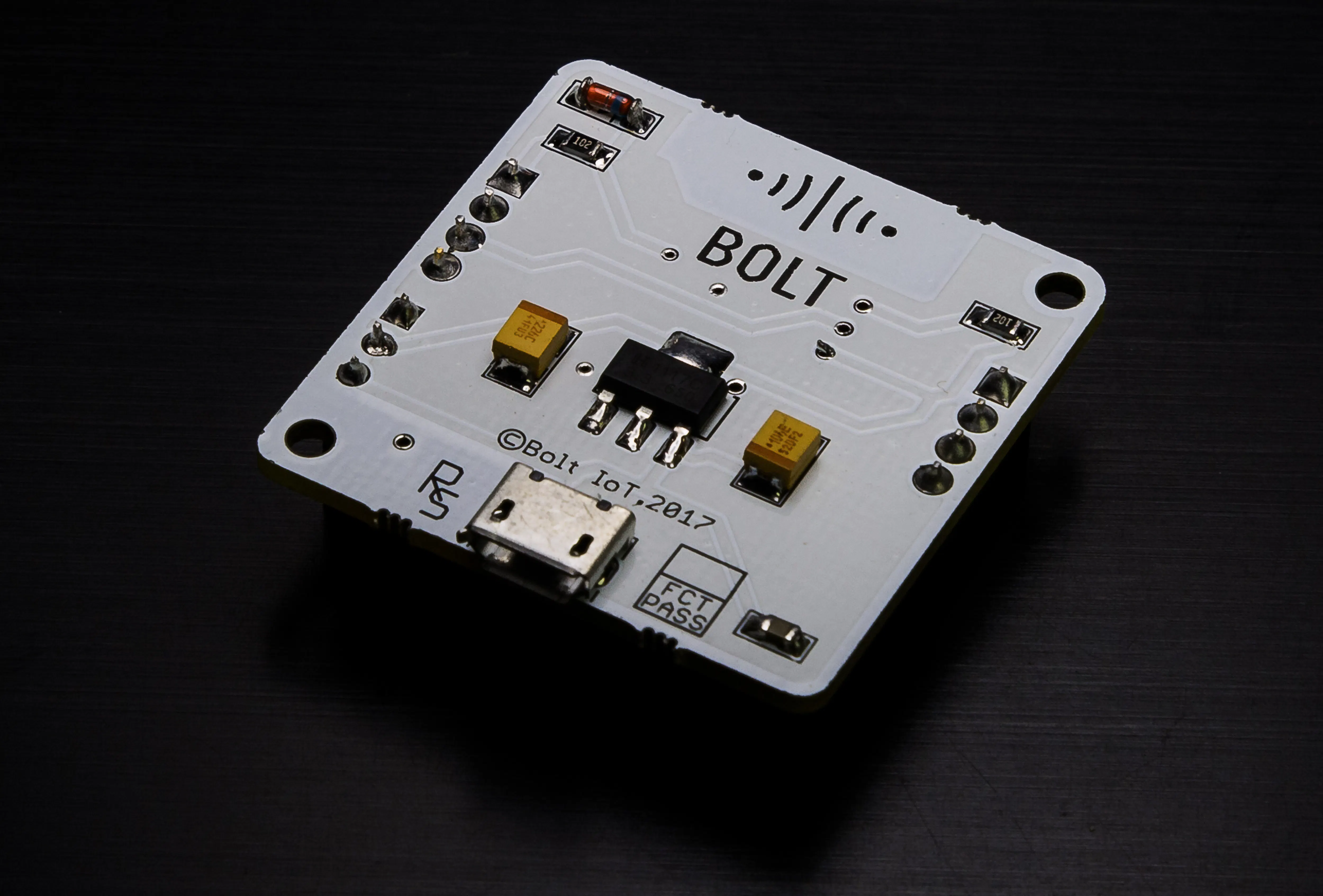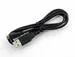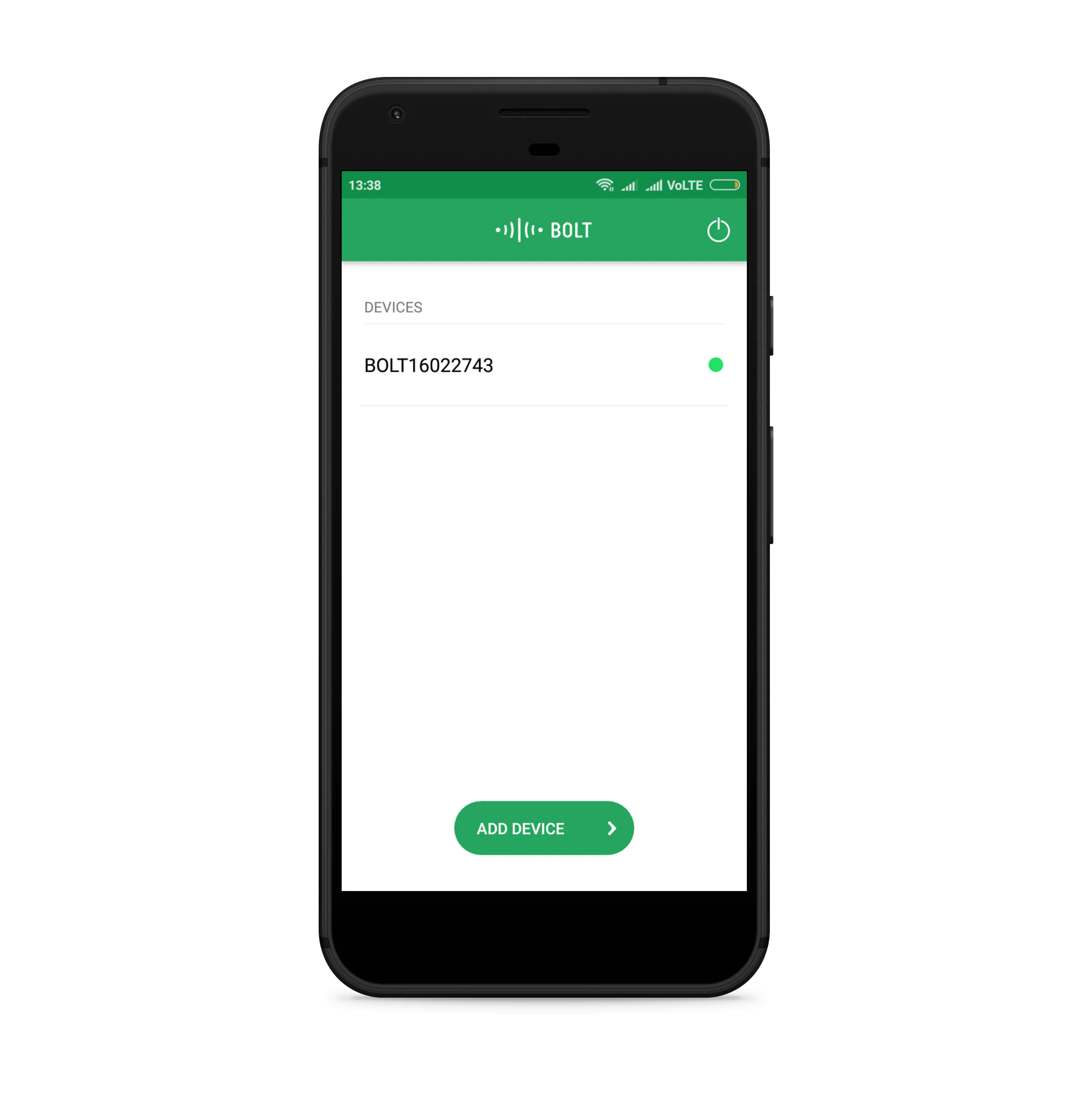Published

# Smart Approach to Fire Prevention and Detection

A cool system that warns us of anomalous temperature, detects fire, sends alert signals using sound and light, notifies us on our phone.

IntermediateFull instructions provided2 hours520## Things used in this project

### Hardware componentsBolt IoT Bolt WiFi Module
×1Temperature Sensor
×1Buzzer
×1LED (generic)
×1×1USB-A to Micro-USB Cable
×1Jumper wires (generic)
×1

### Software apps and online servicesBolt IoT Bolt CloudBolt IoT Android AppSnappy Ubuntu CoreTwilio SMS Messaging API

## Code

### Backend Code for Fire Prevention and Detection System

Python
```#import the necessary libraries
from boltiot import Bolt, Sms
import json, time, math, statistics

#store all credentials related to Twilio and Bolt within double quotes
sid = "" # sid given on Twilio dashboard
auth_token = "" # authorization token given on Twilio dashboard
From = "" #phone number given by Twilio
a = "XXXXXXXXXXXXXXXXX" # your api_key given on Bolt Cloud dashboard
d = "BOLTXXXXXX" # your device_id given on Bolt Cloud dashboard

maxim = 300 #probable analog equivalent of temperature at which fire starts
frame_size = 6 #used for Z-score analysis
mul_factor = 3 #used for Z-score analysis

mb = Bolt(a,d) #create object of Bolt class
sms = Sms(sid,auth_token,From,To) #create object of Sms class
prev_data=[] #stores sensor values

#function to calculate bounds for the temperature sensor using Z-score
def cal_bounds(prev_data,frame_size,mul_factor):
#check if enough data is collected for calculation of Z-score
if len(prev_data)<frame_size :
return None
#deletion of older data
if len(prev_data)>frame_size :
del prev_data[0:len(prev_data)-frame_size]
mean=statistics.mean(prev_data) #calculate mean
var=0
for i in prev_data :
var+= math.pow((i-mean),2) #calculate variance
Zn = mul_factor * math.sqrt(var/ frame_size) #calculate Z-score
Hb= prev_data[frame_size-1]+Zn #calculate upper bound
Lb = prev_data[frame_size-1]-Zn #calculate lower bound
return [Hb,Lb]

#iterate over a loop to sense temperature in theinterval of 10s
while True:
data = json.loads(response) #convert json data to python object
if data['success'] != 1:
print("Error in the data collected: "+data['value']) #print error
time.sleep(10)
continue
print("Sensor value = "+str(data['value']))
bounds = cal_bounds(prev_data,frame_size,mul_factor) #create object of cal_bounds
try:
s_val = int(data['value'])
#check if sensor value exceeds the temperature at which fire starts
if s_val>=maxim:
print("Starting Alarm!")
print("Alarm is ON "+mb.digitalWrite('0','HIGH')) #Buzzer rings
print("Led is ON "+mb.digitalWrite('1','HIGH')) #Led glows
sms.send_sms("Sensor value is " + str(s_val)) #send Twilio alert message
else:
print("Alarm is ON "+mb.digitalWrite('0','LOW')) #Buzzer is silent
print("Led is ON "+mb.digitalWrite('1','LOW')) #Led doesn't glow

#check if enough data has been collected
if not bounds:
print("Need ",(frame_size-len(prev_data))," more data points for Zscore")
prev_data.append(s_val)
continue
try:
#check if sensor value is within the bounds computed by Z-score
if s_val > bounds :
print("Temperature increased suddenly")
#notify anomaly using Twilio
sms.send_sms("Sensor value increased suddenly to " + str(s_val))
prev_data.append(s_val)
except Exception as e:
print("Error in detecting anomaly: ",e) #print error
except Exception as e:
print("Error: ",e) #print error
time.sleep(10)

```